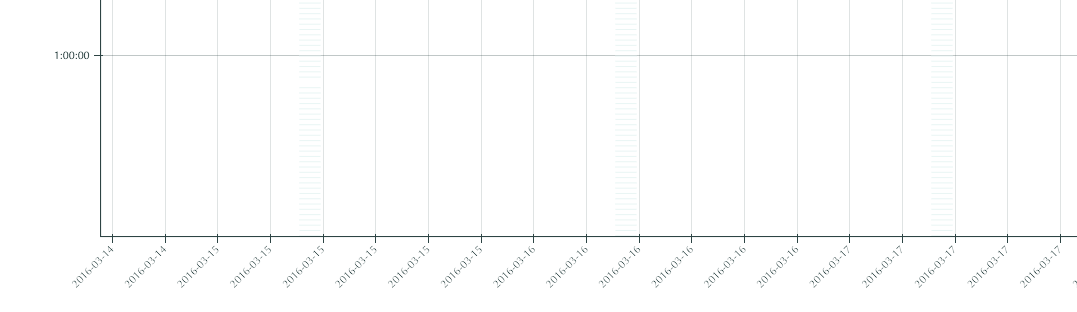# Datetime axis has multiples of a single day using Range1d, DatetimeTickFormatter, DatetimeTicker

Hi,

I’m trying to create a plot where x coordinates are datetime.date objects and y are integers. The code looks like this:

``````p.x_range = Range1d()
p.xaxis.formatter = DatetimeTickFormatter(formats=dict(
hours=["%Y-%m-%d"],
days=["%Y-%m-%d"],
months=["%Y-%m-%d"],
years=["%Y-%m-%d"],
))
``````

p.xaxis.ticker = DatetimeTicker()
p.xgrid.ticker = DatetimeTicker()

# x axis get updated every time d_start changes

def update(…):

``````d_range = pd.date_range(d_start, periods=30, freq='D').date.tolist()
p.x_range.start = d_range
p.x_range.end = d_range[-1]
p.xaxis.ticker = DatetimeTicker(desired_num_ticks=len(d_range), tags=d_range)
p.xgrid.ticker = DatetimeTicker(desired_num_ticks=len(d_range), tags=d_range)
``````
``````
``````
``````The first problem is there are multiples (12 or 24 when zoom in to maximum) ticks of the same day on the axis and the glyph is plotted along only one tick. I want to have one tick a day.
``````
``````Additionally, is there a way to adjust the distance between the first tick on x axis and y axis. At the moment the first tick is at y axis so the tick label is missing as well.
````````````
``````
``````Many thanks in advance!
``````
``````Caroline
``````

Hi caroline,

The reason there are duplicates (I assume) is because the formatter is only showing ticks up to the resolution of days, but when the range only spans a day or two, there are many ticks generated at different hours of the same day.

I guess it really depends on what you are actually trying to achieve:

Do you really only want at most one-tick per day (no intra-day ticks?) Then you could

* reconfigure the .tickers property of DatetimeTicker : https://github.com/bokeh/bokeh/blob/master/bokeh/models/tickers.py#L165

* use a different ticker (maybe SingleIntervalTicker?)

* create a custom ticker subclass

Alternatively, you to want intra-day ticks, just to have them format differently? (e.g. give the day once, and hours on subsequent ticks?) Then maybe:

* use a FuncTickFormatter to specify a different formatting policy

* create a custom tick formatter subclass

Regarding the custom sublass options, the user's guide has a new section of extending bokeh, including some examples specifically around ticking:

Thanks,

Bryan

···

On Jul 15, 2016, at 7:51 PM, Caroline Wang <[email protected]> wrote:

Hi,

I'm trying to create a plot where x coordinates are datetime.date objects and y are integers. The code looks like this:

p.x_range = Range1d()
p.xaxis.formatter = DatetimeTickFormatter(formats=dict(
hours=["%Y-%m-%d"],
days=["%Y-%m-%d"],
months=["%Y-%m-%d"],
years=["%Y-%m-%d"],
))
p.xaxis.ticker = DatetimeTicker()
p.xgrid.ticker = DatetimeTicker()

# x axis get updated every time d_start changes
def update(...):
d_range = pd.date_range(d_start, periods=30, freq='D').date.tolist()
p.x_range.start = d_range
p.x_range.end = d_range[-1]
p.xaxis.ticker = DatetimeTicker(desired_num_ticks=len(d_range), tags=d_range)
p.xgrid.ticker = DatetimeTicker(desired_num_ticks=len(d_range), tags=d_range)

The first problem is there are multiples (12 or 24 when zoom in to maximum) ticks of the same day on the axis and the glyph is plotted along only one tick. I want to have one tick a day.
Additionally, is there a way to adjust the distance between the first tick on x axis and y axis. At the moment the first tick is at y axis so the tick label is missing as well.

Caroline

--
You received this message because you are subscribed to the Google Groups "Bokeh Discussion - Public" group.
To unsubscribe from this group and stop receiving emails from it, send an email to [email protected].
To post to this group, send email to [email protected].

Hi Bryan,

Thank you!

It works when I set ticker property of DatetimeTicker as tickers=[DaysTicker(days=list(range(1, ‘a number here’)))] and normailze the dates value using pd.datetools.normalize_date

···

On Friday, July 15, 2016 at 5:51:59 PM UTC-7, Caroline Wang wrote:

Hi,

I’m trying to create a plot where x coordinates are datetime.date objects and y are integers. The code looks like this:

``````p.x_range = Range1d()
p.xaxis.formatter = DatetimeTickFormatter(formats=dict(
hours=["%Y-%m-%d"],
days=["%Y-%m-%d"],
months=["%Y-%m-%d"],
years=["%Y-%m-%d"],
))
``````

p.xaxis.ticker = DatetimeTicker()
p.xgrid.ticker = DatetimeTicker()

# x axis get updated every time d_start changes

def update(…):

``````d_range = pd.date_range(d_start, periods=30, freq='D').date.tolist()
p.x_range.start = d_range
p.x_range.end = d_range[-1]
p.xaxis.ticker = DatetimeTicker(desired_num_ticks=len(d_range), tags=d_range)
p.xgrid.ticker = DatetimeTicker(desired_num_ticks=len(d_range), tags=d_range)
``````
``````

``````
``````The first problem is there are multiples (12 or 24 when zoom in to maximum) ticks of the same day on the axis and the glyph is plotted along only one tick. I want to have one tick a day.
``````
``````Additionally, is there a way to adjust the distance between the first tick on x axis and y axis. At the moment the first tick is at y axis so the tick label is missing as well.
````````````

``````
``````Many thanks in advance!
``````
``````Caroline
``````